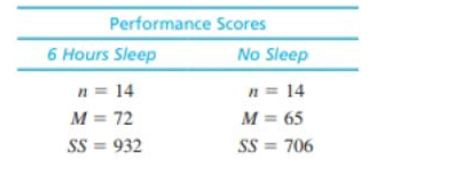Chapter 10, Problem 21PEssentials of Statistics for the B...

8th Edition
Frederick J Gravetter + 1 other
ISBN: 9781133956570

Solutions

Chapter
SectionEssentials of Statistics for the B...

8th Edition
Frederick J Gravetter + 1 other
ISBN: 9781133956570
Textbook Problem

When people learn a new task, their performance usually improves when they are tested the next day, but only if they get at least 6 hours of sleep (Stickgold, Whidbee, Schirmer, Patel, &. Hobson, 2000). The following data demonstrate this phenomenon. The participants learned a visual discrimination task on one day, and then were tested on the task the following day. Half of the participants were allowed to have at least 6 hours of sleep and the other half were kept awake all night. Is there a significant difference between the two conditions? Use a two-tailed test with α = .05.To determine

To check: Whether there is a significant difference between the two conditions using two tailed test with α=0.05.

Explanation

Given info:

The two samples each with size 14 have mean 72 and 65 respectively. The sum of squared difference is 932 and 706 respectively.

Calculation:

Step 1: Null hypothesis is H0:μ1μ2=0; alternate hypothesis is H1:μ1μ20

Step 2: For the given sample, degrees of freedom equals

df=n1+n22=14+142=282=26

For a two-tailed test with α=0.05 and df=26, the critical value (CV) equals t=±2.120

Step 3: t-statistics is calculated as:

t=M1M2(μ1μ2)sM1M2

Pooled variance, sp2:

sp2=SS1+SS2df+df2=932+70613+13=163826

Still sussing out bartleby?

Check out a sample textbook solution.

See a sample solution

The Solution to Your Study Problems

Bartleby provides explanations to thousands of textbook problems written by our experts, many with advanced degrees!

Get Started

Prove the triangle inequality |u+v|u+v.

Calculus: Early Transcendental Functions

Sketch the graph of the set. 40. {(x, y) | x2 + 4y2 4}

Single Variable Calculus: Early Transcendentals

Find the value of the sum. 24. k=08cosk

Single Variable Calculus: Early Transcendentals, Volume I

The slope of the line tangent to the circle x2 + y2 = 100 at the point (6, 8) is: a) 34 b) 34 c) 43 d) 43

Study Guide for Stewart's Single Variable Calculus: Early Transcendentals, 8th

True or False:

Study Guide for Stewart's Multivariable Calculus, 8th